# For Paul Fisher

## Overview of Dissertation

Paul Fisher's (1999) doctoral dissertation (accessible via the LSU Digital Commons) is titled, Nonaxisymmetric Equilibrium Models for Gaseous Galaxy Disks. Its abstract reads, in part:

 Three-dimensional hydrodynamic simulations show that, in the absence of self-gravity, an axisymmetric, gaseous galaxy disk whose angular momentum vector is initially tipped at an angle,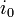$~i_0$, to the symmetry axis of a fixed spheroidal dark matter halo potential does not settle to the equatorial plane of the halo. Instead, the disk settles to a plane that is tipped at an angle,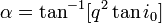$~\alpha = \tan^{-1}[q^2 \tan i_0]$, to the equatorial plane of the halo, where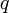$~q$ is the axis ratio of the halo equipotential surfaces. The equilibrium configuration to which the disk settles appears to be flat but it exhibits distinct nonaxisymmetric features. .

All three-dimensional hydrodynamic simulations employ Richstone's (1980) time-independent "axisymmetric logarithmic potential" that is prescribed by the expression,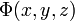$~\Phi(x, y, z)$$~=$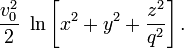$~ \frac{v_0^2}{2}~ \ln\biggl[x^2 + y^2 + \frac{z^2}{q^2} \biggr] \, .$

## Thoughts Moving Forward

Let's continue to examine a collection of Lagrangian fluid elements that are orbiting in an (axisymmetric) oblate-spheroidal potential with flattening "q." But rather than adopting the Richstone potential, we will consider the potential generated inside an homogeneous (i.e., Maclaurin) spheroid whose eccentricity is,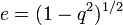$~e = (1 - q^2)^{1 / 2}$, namely,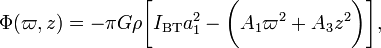$\Phi(\varpi,z) = -\pi G \rho \biggl[ I_\mathrm{BT} a_1^2 - \biggl(A_1 \varpi^2 + A_3 z^2 \biggr) \biggr],$

[ST83], §7.3, p. 169, Eq. (7.3.1)

where, the coefficients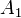$~A_1$,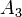$~A_3$, and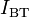$~I_\mathrm{BT}$ are functions only of the spheroid's eccentricity. What does the potential field look like from the perspective of a particle/fluid-element whose orbital angular momentum vector is tipped at an angle,$~i_0$, to the symmetry axis of the oblate-spheroidal potential? Presumably the potential is "observed" to vary with position around the orbit as though the underlying potential is non-axisymmetric. Does it appear to be the potential inside a Riemann S-Type ellipsoid? If so, what values of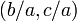$~(b/a, c/a)$ correspond to the chosen parameter pair,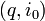$~(q, i_0)$?

Well, let's define a primed (Cartesian) coordinate system whose z'-axis is tipped at this angle,$~i_0$, with respect to the symmetry axis of the oblate-spheroidal potential. Drawing from a discussion in which we have presented a closely analogous methodical derivation of orbital parameters, we have,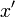$~x'$$~=$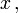$~x \, ,$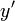$~y'$$~=$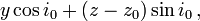$~y \cos i_0 + (z-z_0)\sin i_0 \, ,$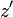$~z'$$~=$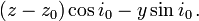$~(z-z_0)\cos i_0 - y\sin i_0 \, .$$~x$$~=$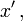$~x' \, ,$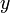$~y$$~=$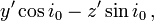$~y' \cos i_0 - z'\sin i_0 \, ,$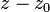$~z-z_0$$~=$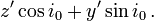$~z'\cos i_0 + y'\sin i_0 \, .$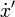$~\dot{x}'$$~=$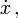$~\dot{x} \, ,$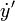$~\dot{y}'$$~=$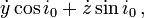$~\dot{y} \cos i_0 + \dot{z}\sin i_0 \, ,$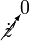$~\cancelto{0}{\dot{z}'}$$~=$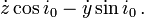$~\dot{z} \cos i_0 - \dot{y}\sin i_0 \, .$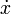$~\dot{x}$$~=$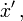$~\dot{x}' \, ,$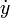$~\dot{y}$$~=$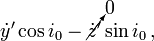$~\dot{y}' \cos i_0 - \cancelto{0}{\dot{z}'}\sin i_0 \, ,$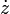$~\dot{z}$$~=$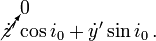$~\cancelto{0}{\dot{z}'}\cos i_0 + \dot{y}'\sin i_0 \, .$

When viewed from this primed frame, the potential associated with a Maclaurin spheroid becomes,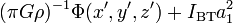$~(\pi G \rho)^{-1} \Phi(x', y', z') + I_\mathrm{BT} a_1^2$$~=$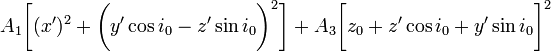$~ A_1 \biggl[ (x')^2 + \biggl(y'\cos i_0 - z' \sin i_0\biggr)^2 \biggr] + A_3 \biggl[ z_0 + z' \cos i_0 + y' \sin i_0 \biggr]^2$$~=$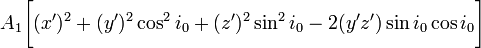$~ A_1 \biggl[ (x')^2 + (y')^2 \cos^2 i_0 + (z')^2 \sin^2 i_0 - 2(y' z')\sin i_0 \cos i_0\biggr]$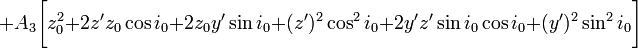$~ + A_3 \biggl[ z_0^2 + 2 z' z_0 \cos i_0 + 2z_0 y' \sin i_0 + (z')^2 \cos^2 i_0 + 2y' z' \sin i_0 \cos i_0 + (y')^2 \sin^2 i_0 \biggr]$$~=$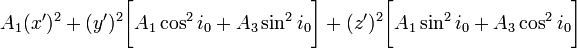$~ A_1 (x')^2 + (y')^2 \biggl[A_1 \cos^2 i_0 + A_3 \sin^2 i_0 \biggr] + (z')^2 \biggl[ A_1 \sin^2 i_0 + A_3\cos^2 i_0 \biggr]$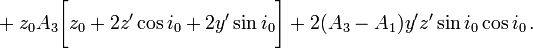$~ + z_0 A_3 \biggl[ z_0 + 2 z' \cos i_0 + 2 y' \sin i_0 \biggr] + 2(A_3 - A_1 )y' z' \sin i_0 \cos i_0 \, .$